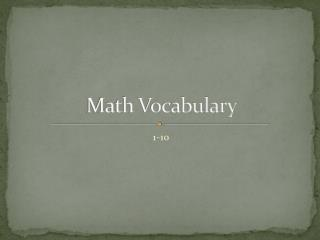DownloadDownload PresentationMath Vocabulary

# Math Vocabulary

Télécharger la présentation## Math Vocabulary

- - - - - - - - - - - - - - - - - - - - - - - - - - - E N D - - - - - - - - - - - - - - - - - - - - - - - - - - -
##### Presentation Transcript

1. Math Vocabulary 1-10

2. Addend = 8 • Number • Part of an addition problem • Numbers added to each other 6 + 2

3. Sum • Number • Answer or result • Addition 4 + 2 = 6

4. Minuend • Number • Subtraction • The number subtracted from • The amount you have to begin with 10 -8 =2

5. Subtrahend • Number • Subtraction • The number subtracted • What you take away 9 10 - = 1

6. Difference • Number • Subtraction • The answer to a subtraction problem • What is left over 1 10 – 9 =

7. Factor • Number • Multiplication • The numbers multiplied by each other • Number of groups • In each (per) group = 9 3 x 3

8. Product • Number • Multiplication • The answer of a multiplication problem • Total (product) 8 2 x 4 =

9. Dividend • Number • Division problem • The number divided into smaller groups • What you begin with 8 8 : 2= 4 /2 = 4

10. Divisor • Number • Division • The number that divides • The number of groups • -or something that does an action 2 2 8: = 4 8/ = 4

11. Quotient • Number • Division • The answer to a division problem • Tells how many in each group 5 5 10: 2 = 10/ 2=